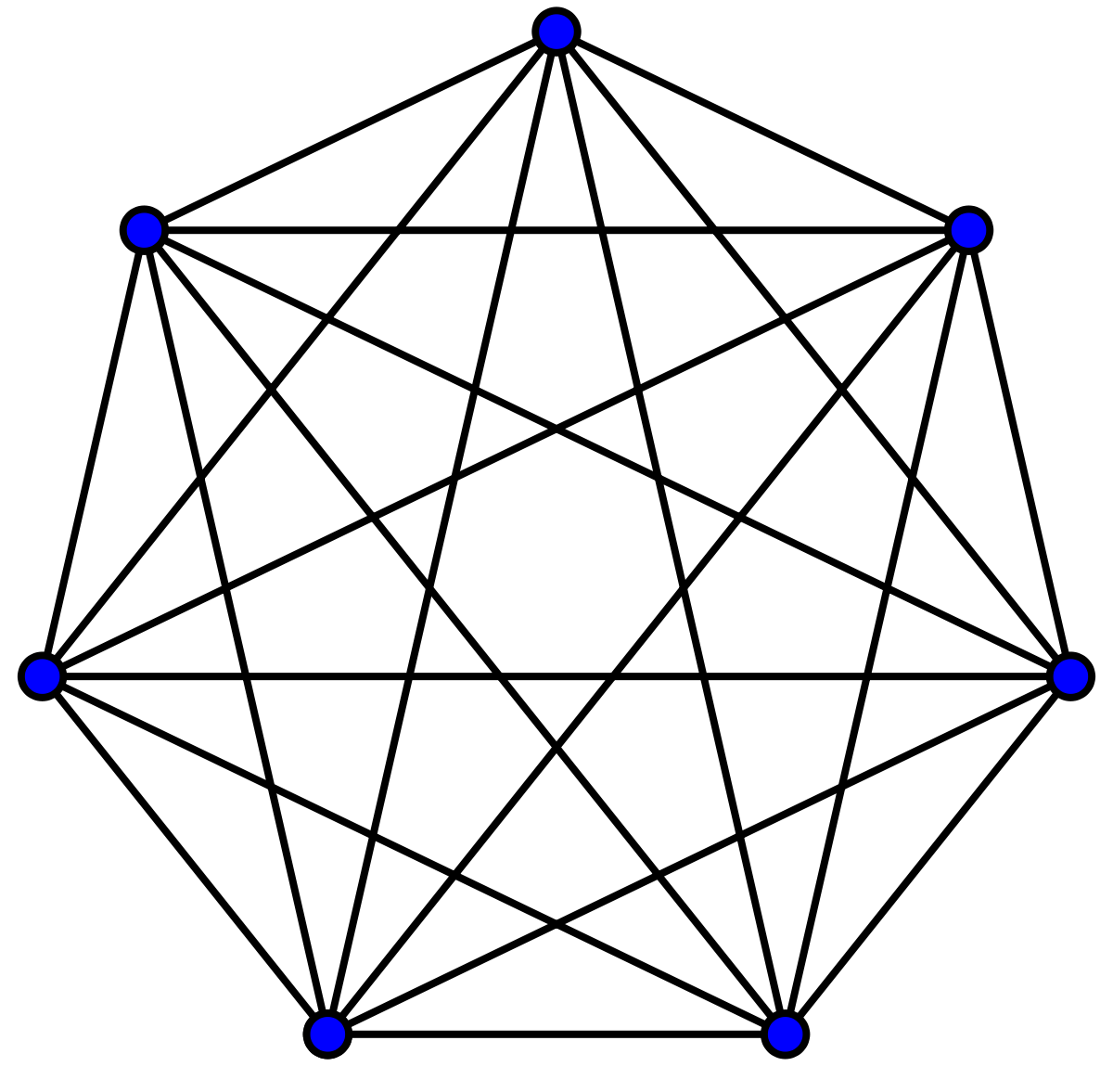# Practice on Toph

Participate in exhilarating programming contests, solve unique algorithm and data structure challenges and be a part of an awesome community.

# Ant-Man And The Polygon

By YouKnowWho · Limits 1s, 512 MB

Ant-Man before escaping from the quantum realm thought about roaming around the realm. And much to his surprise, he found a regular convex polygon with n vertices and side length S. So he decided to walk around it. The polygon was complete i.e. every two vertices were connected by a straight line which are the edges of the polygon(each side is also an edge).He started his walk from a vertex and began to walk through the edges of the polygon. He doesn’t like walking through the same edge more than once but he can visit any vertex more than once. Moreover, he needed to use the edges in such a way that the consecutive visited edges are connected by some vertex. But a few moments later, he found that he can’t visit every edge of the polygon using only one walk in that way. So he decided to remove some of the edges of the polygon so that he can walk around the whole polygon using only one walk. Ant-Man, being a former competitive programmer, thought about removing the edges in such a way that the length of his walk becomes as large as possible. The length of a walk is the sum of lengths of all edges used in the walk and the length of an edge is the euclidean distance between the vertices it connects.A regular polygon is a polygon that is equiangular (all angles are equal in measure) and equilateral (all sides have the same length). All regular simple polygons (a simple polygon is the one that does not intersect itself anywhere) are convex.

## Input

The first line contains an integer t(1 ≤ t ≤ 5000), the number of test cases.

Each of the next t lines contains two integers n and S(3 ≤ n ≤ 1000,1 ≤ S ≤ 100), the number of vertices and the length of the side of the polygon, respectively.

## Output

Output a number, the largest walk length after erasing the optimal edges. The output will be accepted if it has an absolute or relative error at most 10 - 4.

Namely, let’s assume that your answer is a and the answer of the jury is b. The checker program will consider your answer correct if |a-b|/max(1,b) ≤ 10-4 is satisfied.

## Sample

InputOutput
```2
3 3
4 1
```
```9.0000000000
5.8284271247
```

Discussion
Statistics

92% Solution Ratio

SwampFireEarliest, 1M ago

notredFastest, 0.0s

notredLightest, 131 kB

SwampFireShortest, 718B

Submit